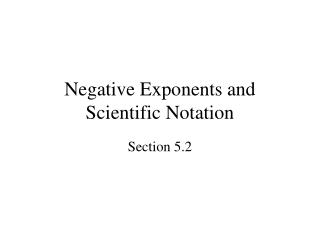DownloadDownload PresentationNegative Exponents and Scientific Notation

# Negative Exponents and Scientific Notation

Télécharger la présentation## Negative Exponents and Scientific Notation

- - - - - - - - - - - - - - - - - - - - - - - - - - - E N D - - - - - - - - - - - - - - - - - - - - - - - - - - -
##### Presentation Transcript

1. Negative Exponents and Scientific Notation Section 5.2

2. Overview • Negative Integers as Exponents • Scientific Notation • Multiplying, Dividing, and Significant Digits • Problem Solving Using Scientific Notation

3. Negative Integers as Exponents • For any real number a that is nonzero:

4. Negative Integers as Exponents • For any real number a and b that is nonzero:

5. Negative Integers as Exponents • For any real number a and b that is nonzero:

6. Scientific Notation • Useful way of writing very large or very small numbers that frequently occur in science: Where N is at least 1 but less than 10; N is expressed in decimal notation, and m is an integer

7. Scientific Notation • Mass of earth: 6.0 × 1024 kilograms = 6,000,000,000,000,000,000,000,000 kg • Mass of a hydrogen atom: 1.7 × 10-24 grams = 0.0000000000000000000000017 g

8. Scientific Notation • One thousand = 1,000 = 103 • One million = 1,000,000 = 106 • One billion = 1,000,000,000 = 109 • One trillion = 1,000,000,000,000 = 1012 • One quadrillion = 1,000,000,000,000,000 = 1015 • One googol = 10100 • One googolplex = 10googol • (No such thing as a “Jillion” or “Bazillion”)

9. Scientific Notation • Converting scientific notation to decimal notation: • 4.52 × 102 = 4.52 × 100 = 452 • 4.52 × 101 = 4.52 × 10 = 45.2 • 4.52 × 100 = 4.52 × 1 = 4.52 • 4.52 × 10-1 = 4.52 × 0.1 = 0.452 • 4.52 × 10-2 = 4.52 × 0.01= 0.0452

10. Multiplying, Dividing, and Significant Digits • Significant Digits 5.12 × 103 Three significant digits 5.1 × 103 Two significant digits • More significant digits= more precise measurement

11. Multiplying, Dividing, and Significant Digits • When multiplying or dividing, result should be rounded so it has same number of significant digits as measurement with fewest significant digits. Rounding should be performed at the end of the calculation • (3.1 × 10-3 mm)(2.45 × 10-4 mm) = 7.595 × 10-7 mm2 = 7.6 × 10-7 mm2

12. Multiplying, Dividing, and Significant Digits • When adding or subtracting, result should be rounded so it has same number of decimal places as measurement with fewest decimal places. Rounding should be performed at the end of the calculation • (1.6354 × 104 km) + (2.078 × 104 km) = 3.7134 × 104 km = 3.713 × 104 km

13. Problem Solving Using Scientific Notation • 5 exabytes of information (1 exabyte = 1012 megabytes) generated by worldwide population of 6.3 billion people • What is average number of megabytes generated per person